Courses

# Important Questions: Circles

## 10 Questions MCQ Test Mathematics (Maths) Class 10 | Important Questions: Circles

Description
This mock test of Important Questions: Circles for Class 10 helps you for every Class 10 entrance exam. This contains 10 Multiple Choice Questions for Class 10 Important Questions: Circles (mcq) to study with solutions a complete question bank. The solved questions answers in this Important Questions: Circles quiz give you a good mix of easy questions and tough questions. Class 10 students definitely take this Important Questions: Circles exercise for a better result in the exam. You can find other Important Questions: Circles extra questions, long questions & short questions for Class 10 on EduRev as well by searching above.
QUESTION: 1

Solution:
QUESTION: 2

### In the given figure, AB and AC are tangents to the circle with centre O such that ∠BAC = 40°, then ∠BOC is equal to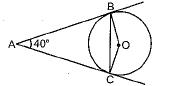Solution:

In quadrilateral ABOC
∠ABO + ∠BOC + ∠OCA + ∠BAC = 360°
⇒ 90° + ∠BOC + 90° + 40° = 360°
⇒ ∠BOC = 360° - 220° = 140°

QUESTION: 3

### In Fig. 8.5, PQ is a chord of a circle and PT is the tangent at P such that ∠QPT = 60°. Then ∠PRQ is equal to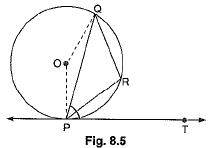Solution:
QUESTION: 4

In the given figure, point P is 26 cm away from the centre O of a circle and the length PT of the tangent drawn from P to the circle is 24 cm. Then the radius of the circle is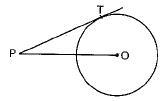Solution: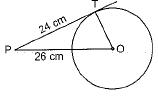∵ OT is radius and PT is tangent
∴ OT ⊥ PT
Now, in ΔOTP,
OP2 = PT2 + OT2
⇒ 262 = 242 + OT2
⇒ 676 - 576 -OT2
⇒ 100 = OT2 ⇒ 10 cm = OT

QUESTION: 5

In Fig. 8.6, if PA and PB are tangents to the circle with centre O such that ∠APB = 50°, then ∠PAB is equal to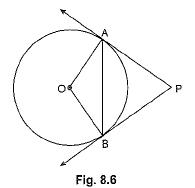Solution:
QUESTION: 6

A line through point of contact and passing through centre of circle is known as

Solution:
QUESTION: 7

The length of the taragent drawn from a point 8 cm away from the centre of a circle of radius 6 cm is

Solution: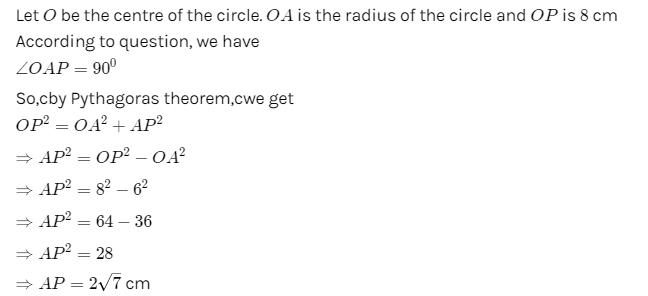QUESTION: 8

C (O, r1) and C (O, r2) are two concentric circles with r1 > r2. AB is a chord of C (O, r1) touching C (O, r2) at C then

Solution: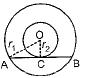∵ AB touches
C(O, r2)
∴ OC ⊥ AB
Also, perpendicular from the centre to a chord bisects the chord.
∴ AC = BC

QUESTION: 9

In Fig. 8.9, if ∠AOB = 125°, then ∠COD is equal to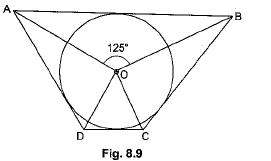Solution:
QUESTION: 10

Two parallel lines touch the circle at points A and B respectively, If area of the circle is 25πcm2, then AB is equal to

Solution:

Let radius of circle = R
∴ πR2 = 25π
⇒ R = 5cm
∴ Distance between two parallel tangents
= diameter = 2 x 5 = 10 cm.